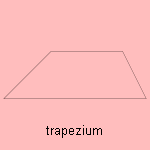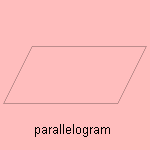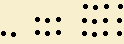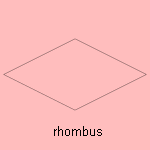## line

last updated: 2006-03-14

There are many quadrilaterals to distinguish. The tangent quadrilateral and the cyclic quadrilateral are special cases of the tangent polygon and the cyclic polygon.
For the tangent quadrilateral the opposite sides are of equal length. A cyclic quadrilateral for which the product of the opposite sides is the same, is called a harmonic quadrilateral.A trapezium or trapezoid is a quadrilateral with only two parallel sides. The trapezoid rule gives a way to find the area under a give curve.
When the non parallel sides are equal in length, the figure is a isosceles trapezium. We see it in the trapezium curve - a repetition of trapeziums - and in the so-called trapezoid screw-thread.The parallelogram is a quadrilateral for which the opposite sides are parallel. It can be seen as representing the vector sum of two variables with length and direction (e.g. a force), resulting in the diagonal of the parallelogram.
Anna Isabella Milbanke (who has been married to Lord Byron) had as nickname the 'princess of the parallelograms', for her interest in mathematics.

A crystal lattice in two dimensions is build of a repeating structure in two directions, forming a parallelogram. This shows that a plane can be filled with parallelograms.

The rectangle has only right 1) angles. It is a very handy form. How many objects have a rectangular form: the pages of a book, this web page, the room I am sitting in, the piece of  ground of our house, the window I am looking through, the doors in my house: all rectangles. So while building a house many right angles have to be constructed. We can make this construction easier when we use the Pythagoras theorem, and make windows in a ratio of 3 to 4 for the height related to the length. The right angle is easy to construct by pacing a diagonal of 5 units. In Holland in old farms such windows can be seen.
Also many other object have this rectangular form: writing paper, postcards, football fields, paintings, tables, shelves. Package material as boxes consists often of rectangles, what makes piling up more easy.

In perspective the rectangle seems deformed, for the eye. In such a way that parallel lines - in the direction of the observer - meet each other in the so-called vanishing-point. Our brains can compensate for this deformation, and we will often be able to recognize a rectangle.
In a perspective view the scale is not constant, so that is difficult to measure and   and count. An oblique projection, where a rectangle is shown as a parallelogram, is easier for this purpose.

The golden section is a ratio between the sides of a rectangle, that looks quite natural. We define this figure so that when you cut a square from the rectangle, the resulting rectangle is similar to the original rectangle 2) . We call it the golden rectangle.

The rectangular numbers 2, 6, 12, 20 and so on can be defined analogous to the triangular numbers:and so on.The rectangle is repeated in the rectangular curve.

A rhombus is an oblique square: the four sides are equal in length, but there are no right angles.
In Dutch a rhombus is called a 'ruit', a word which originated from the medieval 'ru(y)te', which also denoted the similar formed plant. A strange phenomenon is that some rectangular objects have the rhombus in their Dutch name: this is the case for the words for: pane, squared paper and tartan. The checker 'ruit' is a real rhombus, as is the 'wyber' (liquorice) and the Renault logo.

When the sides are in pairs equal in length, we see a kite.The square is the regular quadrilateral. Of all rectangles with equal circumference the square has the maximum area (what has been proved already by Euclid, about 300 BC).
Lifting a square to three dimensions gives a cube.

The square numbers 1, 4, 9, 16 and so on, were for the Pythagoras group the symbol of justice. The quadratic equation and the quadratic root are also based on the power two.
A magic square is a matrix of numbers, where the sum of rows, columns and diagonals has the same result.

A curve of constant width is a curve that makes contact with all four sides, when rotated in a square. Such a curve is also known as a roller.
Examples are:

A pentamino is a curve formed by joining squares together.

notes

1) rectus (Lat.) = right.